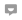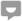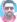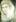# Theory of Relativity Made Simple

Knoji reviews products and up-and-coming brands we think you'll love. In certain cases, we may receive a commission from brands mentioned in our guides. Learn more.
Einsteins' Theory of Special Relativity is explained in a simple manner for the interested person.

Every educated person has heard the phrase "Theory of Relativity". However, only few people understand it because it is an advanced concept of physics.

History: Motion and laws of motion were one of the first phenomena studied in detail in modern western science. Their desire was to find the fundamental laws that governed motion so as to express them in terms of precise mathematical formulation.  Galileo Galilee was one of the first to succeed when he proposed his laws of motion, and expressed them in terms of three mathematical expression. These laws and equations are taught at school level even today, and they form an essential framework to understand motion.

Application of the equations of motion gives excellent result for all terrestrial and even celestial moving bodies. However, in 1887 by Albert Michelson and Edward Morley performed a famous experiment on the velocity of light where the equations of motion broke down. The result was totally different from predication. After numerous repetitions it was confirmed that the the experimental results were right and that the equations of motion do not hold in the case of light. This observation perplexed the entire world of physics.

Albert Einstein postulated that the speed of light does not vary with observer, and came up with a mathematical explanation of the the phenomenon in 1905. This explanation was eventually labelled the Special Theory of Relativity, or Relativity in short.

The Special Theory: Since the mathematical derivation given by Einstein gave good result for almost all observations and experiments related to the speed of light and related phenomenon, the theory gained popularity. The application of the theory helps to predict phenomena that take place very near or at the speed of light.

For example, the theory predicts that as the velocity of a moving body approaches the speed of light its mass begins to increase and will become infinite. Since a body of infinite mass needs infinite energy to accelerate it further, and since there is nothing like infinite energy, the theory predicted that moving bodies will never exceed the speed of light.The mathematical derivations that Einstein also proposed that time should be considered as a dimension of space in addition to length, breadth, and height. When all of this is put together, another deduction is that mass and energy are two forms of one and the same entity. This relationship is expressed in terms of the equation shown on the left. E is energy, m is mass and c is the velocity of light. This also implies that if a mass m is completely converted into energy, it would be equal to the mass multiplied by the square of the velocity of light.

Since the velocity of light is 300,000 kilometer per second, one can imagine the huge amount of energy that will be released when one gram of matter is converted into energy. This is the principle behind atomic energy and atom bombs. In atomic energy plants mass is converted into heat energy in a controlled manner and the heat is harnessed to produce electricity. In atom bomb a huge mass is converted into energy within a microscopic period of time and the heat thus released destroyers everything around it.

Conclusion: Some people claim that Einstein proved that everything was relative. On the contrary, what he stated was that the velocity of light is constant. The term Relativity is used for it because of considerations other than relativism of things. The theory has had numerous theoretical and practical consequences, the atomic energy and bomb being only one.

My Science Simplified Factoids:Can Protons Or Neutrons Be Split

Is Time-travel A Fact?

Are Coprolites Fossil Dung?

How To Clean Old Silver Coins

Picture Credits: Albert Einstein, Mass-Energy Equation (GFDL Copyright)Stephanie Harrison
0
Posted on Jan 8, 2012David Young
0
Posted on Nov 18, 2011Guest
Posted on Nov 7, 2011Danny Franklin
0
Posted on Oct 22, 2011Guest
Posted on Aug 24, 2011Guest
Posted on Aug 2, 2011Marcos Antonio Moreno de Oliveira
0
Posted on Jul 16, 2011benjamin abraham
0
Posted on Jul 4, 2011Jeff Grant
0
Posted on Apr 14, 2011Elijah Baba
0
Posted on Mar 17, 2011Guest
Posted on Mar 15, 2011Mukhia Gideon
0
Posted on Mar 6, 2011lucia anna
0
Posted on Dec 14, 2010Nick Davaine
0
Posted on Jul 27, 2010Mark Schmidt
0
Posted on Jul 20, 2010Ed Molina
0
Posted on May 26, 2010